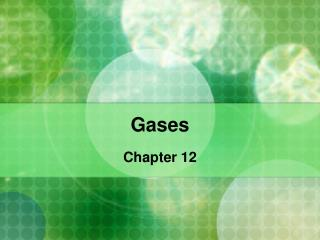DownloadDownload PresentationGases

Gases

Télécharger la présentationGases

- - - - - - - - - - - - - - - - - - - - - - - - - - - E N D - - - - - - - - - - - - - - - - - - - - - - - - - - -
Presentation Transcript

1. Gases Chapter 12

2. Gases • Properties: • Gases are fluids because their molecules/atoms can flow • Gases have low density • Highly compressible – their volume can be reduced • Gases will completely fill their container

3. Gases • Gas Pressure • In the atmosphere, gas molecules collide with Earth’s surface to create atmospheric pressure.

4. Gases • As you descend toward earth, the atmosphere is denser and the pressure is higher • When flying your ears may “Pop” due to the change in pressure

5. Gases • Standard Temperature and Pressure • Short form: STP • Scientists have specified a set of standard conditions when studying the effects of changing temperature and pressure • Stands for:: • 0 degrees C • 1 atm

6. Gases • How to convert between pressure units Use dimensional analysis Ex: Convert the pressure of 1.000 atm to mm of mercury Conversion factor: 101325 Pa and 1 mm Hg 1 atm 133.322 Pa Calculation: 1.000 atm x 101325 Pa x 1 mm Hg = 760 mm Hg 1 atm 133.322 Pa

7. Gases – Converting Between Units

8. Gases • Practice • The critical pressure of carbon dioxide is 72.7 atm. What is this value in units of pascals? • The vapor pressure of water at 50 deg C is 12.33kPa. What is this value in mm of Hg?

9. Gases – Kinetic Molecular Theory • A model used to predict gas behavior • Using the following animation, we will determine the main points of the theory • http://www2.biglobe.ne.jp/~norimari/science/JavaApp/Mole/e-gas.html • http://www.chm.davidson.edu/ChemistryApplets/KineticMolecularTheory/PT.html

10. Gases • Pressure-Volume Relationships Boyle’s Law Based on the following facts: 1. Gases can be compressed 2. Gases exert pressure Boyle found that: As volume decreases, the concentration, and therefore the pressure, increases

11. Gases As volume decreases, the concentration, and therefore the pressure, increases

12. Gases • Boyle’s Law States: • For a fixed amount of gas at a constant temperature: as the volume of the gas decreases the pressure increases • We can use the following equation to calculate changes in pressure or volume

13. Gases • A graph of this equation looks like:

14. Boyle’s Law http://www.grc.nasa.gov/WWW/K-12/airplane/aboyle.html Animation of Boyle’s Law

15. Gases

16. Gases • Boyle’s Law • Example: • A given sample of gas occupies 523 mL at 1.00 atm. The pressure is increased to 1.97 atm, while the temperature remains the same. What is the new volume of gas?

17. Gases • Temperature-Volume Relationships • Charles’ Law • For a fixed amount of gas at a constant pressure, the volume of a gas increases as the temperature of the gas increases

18. For a fixed amount of gas at a constant pressure, the volume of a gas increases as the temperature of the gas increases

19. Charles’ Law Formula: Remember! ALWAYS USE KELVIN when dealing with temperature in gas laws! All °C temperatures MUST be converted to Kelvin!!!

20. Charles’ • http://wright.nasa.gov/airplane/aglussac.html Animation of Charles’ Law

21. Gases • Example: • A balloon is inflated to 665 mL volume at 27 deg C. It is immersed in a dry-ice bath at -78.5 deg C. What is its new volume, assuming the pressure remains constant?

22. Gases • Temperature-Pressure Relationships • Gay-Lussac’s Law: • The pressure of a gas at a constant volume is directly proportional to the absolute temperature (temperature in Kelvin)

23. Gay-Lussac’s Law Formula: At constant volume: P1 = P2 T1 T2

24. Gay-Lussac’s Law • http://www.marymount.k12.ny.us/marynet/06stwbwrk/06gas/1amcslussac/amcsgaylussac.html • Animation of Gay-Lussac’s Law

25. Gases – Avogadro’s Law • In 1811, Avogadro proposed that equal volumes of all gases, under the same conditions, have the same number of particles. • http://www.marymount.k12.ny.us/marynet/06stwbwrk/06gas/2lpimavogdro/2lpimavogadro.html

26. Gases – Avogadro’s Law • We know volume of a gas can change with temperature and pressure, but what about the number of molecules? • Through Avogadro’s observations, the following has been defined: • 1 mole of any gas at STP (0°C and 1 atm) occupies 22.41 L • The mass of 22.41L at STP is the Molecular Mass of the gas

27. Gases - Combined Gas Law • When you take Boyles, Charles’, Gay-Lussac’s, and Avogadro’s Laws and combine them, you get the COMBINED GAS LAW This law is used to solve problems where pressure, volume and temperature of a gas vary with a constant molar quantity of the gas

28. Gases – Combined Gas Law • Example: • A sample of hydrogen gas has a volume of 65.0 mL at a pressure of 0.992 atm and a temperature of 16 deg C. What volume will be hydrogen occurpy at 0.984 atm and 25 deg C?

29. Gases – Dalton’s Law of Partial Pressures • John Dalton showed that in a mixture of gases, each gas exerts a certain pressure as if it were alone with no other gases with it. • This is called “partial pressure”

30. Gases – Dalton’s Law of Partial Pressures

31. Gases – Dalton’s Law of Partial Pressures